# NCERT CBSE Notes for Class 9 Science physics Chapter 12 SOUND

NCERT CBSE Notes For Class 9 Science Physics Chapter 12 SOUND Download Pdf

Chapter 12 NCERT CBSE Notes for Class 9 Science physics SOUND

## NCERT CBSE Notes for Class 9 Science physics Chapter 12 SOUND 1. Production of Sound

Sound is produced due to the vibration of objects. Vibration is the rapid to and fro motion of an object.

Vibrating objects are the source of all sounds Irregular, chaotic vibrations produce noise Regular, controlled vibration can produce music All sound is a combination of pure frequencies
A stretched rubber band when plucked vibrates and produces sound.

2. Propagation of Sound

When an object vibrates, the particles around the medium vibrate. The particle in contact with the vibrating object is first displaced from its equilibrium position

The disturbance produced by the vibrating body travels through the medium but the particles do not move forward themselves.

A wave is a disturbance which moves through a medium by the vibration of the particles of the medium. So sound is considered as a wave.Sound waves Require medium for transmission.Sound waves are called mechanical waves. When a vibrating object moves forward, it pushes and compresses the air in front of it forming a region of high pressure called compression (C). When the vibrating object moves backward, it forms a region of low pressure called rarefaction (R).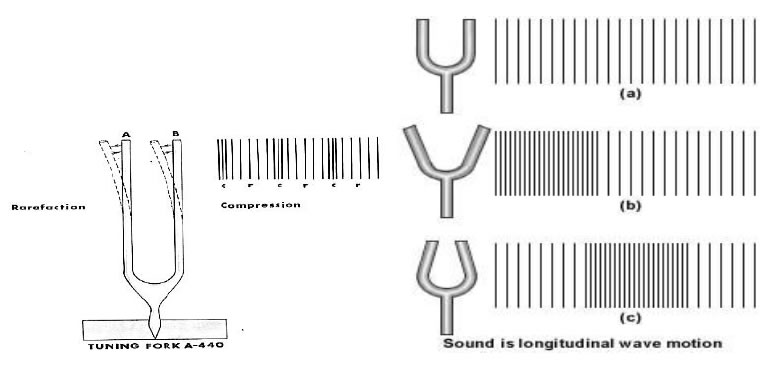A vibrating object producing a series of compressions (C) and rarefaction (R)
In these waves the particles move back and forth parallel to the direction of propagation of the disturbance. Such waves are called longitudinal waves.

There is another kind of waves called transverse waves. In these waves the particles oscillate up and down perpendicular to the propagation of the direction of disturbance.
Sound propagates in a medium as a series of compressions (C) and rarefactions (R).
Compressions are the regions of high pressure and density where the particles are crowded and are represented by the upper portion of the curve called crest.
Rarefactions are the regions of low pressure and density where the particles are spread out and are represented by the lower portion of the curve called trough

Characteristics of a sound wave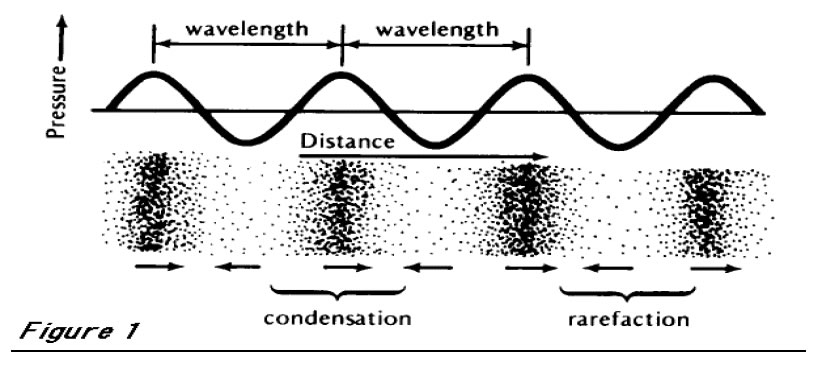Frequency of sound wave

The number of oscillations per unit time is called the frequency of the sound wave.
It is represented by the symbol ٧ (Greek letter nu). Its SI unit is hertz (Hz)

Time period of sound wave
Frequency and time are represented as follows:-

٧ for one oscillation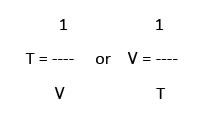Amplitude of sound wave

The amplitude of sound wave is the height of the crest or tough.
It is represented by the letter A. The SI unit is the same as that of density or pressure.Wavelength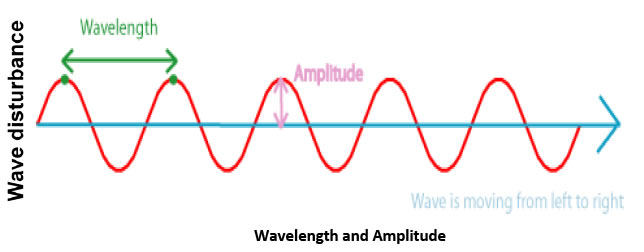The wavelength is the distance between the "crests" of two waves that are next to each other. The amplitude is how high the crests are

Pitch and loudness of sound

The pitch of sound (shrillness or flatness) depends on the frequency of vibration.

If the frequency is high, the sound has high pitch and if the frequency is low, the sound has low pitch
Speed of sound

The speed of sound is more in solids, less in liquids and least in gases.

The speed of sound also depends on the temperature of the medium. If the temperature of the medium is more, the speed of sound is more

3. Reflection of Sound

Sound gets reflected at the surface of a solid or liquid and follows the laws of reflection.

i) The angle of incidence is equal to the angle of reflection.

ii) The incident ray, the reflected ray and normal at the point of incidence all lie in the same plane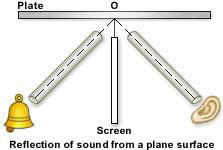4. Echo

If we shout or clap near a reflecting surface like tall building or a mountain, we hear the same sound again. This sound which we hear is called echo. It is caused due to the reflection of sound.

To hear an echo clearly, the time interval between the original sound and the echo must be at least 0.1 s.

Since the speed of sound in air is 344 m/s, the distance travelled by sound in 0.I s = 344 m/s x 0.1 s = 34.4 m

So to hear an echo clearly, the minimum distance of the reflecting surface should be half this distance that is 17.2 m.

Reverberation

Echoes may be heard more than once due to repeated or multiple reflections of sound from several reflecting surfaces. This causes persistence of sound called reverberation. In big halls or auditoriums to reduce reverberation, the roofs and walls are covered by sound absorbing materials like compressed fibre boards, rough plaster or draperies.

5. Uses Of Multiple Reflection Of Sound

Megaphones, horns, musical instruments like trumpets, etc. are deigned to send sound by multiple reflection in a particular direction without spreading in all directions.

ii) Doctors listen to sounds from the human body through a stethoscope. The sound of heartbeat reaches the doctor’s ears by multiple reflection.

iii) Generally the ceilings of cinema halls and auditoriums are curved so that sound after multiple reflection reaches all parts of the hall. Sometimes a curved sound board is placed behind the stage so that sound after multiple reflection spreads evenly across the hall.

6. Range of Hearing

Human beings can hear sound frequencies between 20 Hz and 2000 Hz

Sound whose frequency is less than 20 Hz is called infrasonic sound
Sound whose frequency is more than 2000 Hz is called ultrasonic sound

7. Uses of ultrasonic sound

Ultrasonic sound is used to clean objects like electronic Components, used to detect cracks in metal blocks, used in ultra sound scanners for getting images of internal organs of the human body used to break small stones formed in the kidneys into fine grains.

8 Sonar

It is a device which uses ultrasonic waves to measure distance, direction and speed of underwater objects. The distance of the object can be calculated by knowing the speed of sound in water and the time taken between the transmission and reception of ultrasound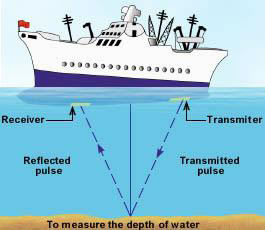9.Structure  of the human ear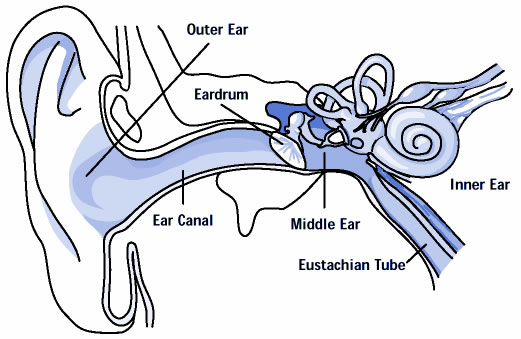The sound waves passes through the ear canal to a thin membrane called eardrum. The eardrum vibrates. The vibrations are amplified by the three bones of the middle ear called 130 hammer, anvil and stirrup. Middle ear then transmits the sound waves to the inner ear. The brain then interprets the signals as sound.

### QUESTION BANK

One mark questions

1. What do you understand by sound waves?

2. Give an example to show that sound travels at a finite speed.

3. Is sound wave longitudinal or transfer.

4. Name two quantities that vary periodically at a place in air as a sound wave travels through it .

5. An airplane produces a sound wave with frequency of 5 KHz and wavelength 30 m. In how much time would the sound wave cover the distance of 4 Km?

6. With the help of a diagram show how longitudinal waves like sound waves travel in air.

7. With the help of a diagram show how longitudinal waves like sound waves travel in air.

8. Can we hear the ringing of a mobile phone placed in a vacuum chamber?

9. Can two astronauts talk on moon a they does on the surface of the earth?

Two marks questions

1. Explain how echoes are used by bats to judge the distance of an obstacle?

2. State the special properties of ultrasound that make it useful to us .In general, how these properties are utilized.

3. Why is soft furnishing avoided in concert halls?

4. Draw a diagram depicting low pitched sound and high pitched sound and write main difference between the two?

5. Distinguish between longitudinal and transverse waves.

6. An explosion takes place at the moon. After what time would it be heard at the earth?

Give one example each.

Three marks questions

1. Two sources A and B vibrate with the same amplitude. They produce sounds of frequencies 1 kHz and 30 kHz respectively. Which of the two waves will have greater power?

2. Find the time period of the source of a sound wave whose frequency is 400Hz.

3. A sound wave travels at a speed of 340m/s. If its wavelength is 2 cm, what is the frequency of the wave? Will it be in the audible range?

4. The grandparents and parents of a two year girl are playing with her in a room. A sound source produces a 28—kHzsound.who in the room is most likely to hear the sound?

Five marks questions

1. Sound cannot travel in vacuum. Describe an experiment to demonstrate this.

2. With the help of a diagram describe how compression and rarefaction pulses are produced in air near a source of sound. 3. Explain briefly how a flaw in a mental component can be detected using ultrasound?

4. Explain the working and application of SONAR.

5. A monkey drops a coconut from the top of a tree. He hears the sound of the coconut hitting the ground 2.057 seconds after dropping it .If the monkey was 19.6 metres above the ground, what is the speed of sound in air?(take g = 9.8m/s2.

6. Draw a neat diagram of human ear. Explain the function of various parts.

What have you learnt Longitudinal waves:

Those in which the direction of vibration is the same as their direction of propagation. So the movement of the particles of the medium is either in the same or in the opposite direction to the motion of the wave. Exemple: sound waves, what changes in this case is the pressure of the medium (air, water or whatever it be). Transverse waves: The oscillations occur perpendicularly to the direction of energy transfer.

Exemple: a wave in a tense string. Here the varying magnitude is the distance from the equilibrium horizontal position A general property of waves is that their speed relative to medium depends on the properties of medium but is independent of the motion of the source of waves. If the observer is in motion with respect to the medium, the velocity of wave propagation relative to the observer wil be different. A remarkable exception is encountered in the case of light

PROPERTIES

Frequency -
Wavelength -
Period -
Amplitude -
Intensity -
Speed -
Direction Perception of Sound For humans, hearing is limited to frequencies between about 20 Hz and 20000 Hz, with the upper limit generally decreasing with age.

### NCERT CBSE Notes for Class 9 Science physics Chapter 12 SOUND (ncerthelp.com)

KEY LEARNING :

Vibration -
repetitive back and forth motion Periodic motion -
a motion that repeats itself Mechanical waves require medium for propagation Waves move through medium but medium remains in place Longitudinal waves-Vibration direction parallel to wave propagation direction Particles in medium move closer together/farther apart .Example: sound waves Gases and liquids -
support only longitudinal waves Transverse waves-
Vibration direction perpendicular to wave propagation direction .Example: plucked string Solids -
support both longitudinal and transverse waves Sound waves Require medium for transmission 1. Sound is a wave motion, produced by a vibrating source.

2. A medium is necessary for the propagation of sound waves.

3. Sound is a longitudinal wave in which the particles of medium move along the direction of motion of wave.

4. The part or region of a longitudinal wave in which the density of the particles of the medium is higher than the normal density is known as compression.

5. The part or region of a longitudinal wave in which the density of the particles of the medium is lesser than the normal density is called a rarefaction.

6. The point of maximum positive displacement on a transverse wave is known as crest.

7. The point of maximum negative displacement on a transverse wave is known as through.

8. A wave or short duration which is confined to a small portion of a medium at any given time is known as a pulse.

9. The maximum displacement of particles of the medium from their mean positions during the propagation of a wave is known as amplitude of the wave.

10. The distance traveled by a wave in one second is called wave velocity. It depends upon the nature of the medium through which it passes.

11. The speed of sound depends primarily on the nature and the temperature of the transmitting medium.

12. Sound travels faster in solids than in air. The speed of sound in solids is much more than the speed of sound in liquids or gases.

13. The distance between two consecutive compressions or two consecutive rarefactions is called the wavelength.

14. Frequency is defined as the number of oscillations per second.

15. The time taken by the wave for one complete oscillation of the density or pressure of the medium is called the time period, T.

16. How the brain interprets the frequency of an emitted sound is called the pitch of sound.

17. Loudness is the degree of sensation of sound produced .

18. Sound properties such as pitch, loudness and quality are determined by the corresponding wave properties.

19. Sound gets reflected and follows the same law as the reflection of light.

20. The persistence of sound due to repeated reflection and its gradual fading away is called reverberation of sound.

21. Echo is a repetition of sound due to the reflection of original sound by a large and hard obstacle.

22. The audible range of hearing for average human beings is in the frequency range of 20 Hz – 20 kHz.

23. The amount of sound energy passing each second through unit area is called the intensity of sound.

24. Sound of frequency less than 20 Hz is known as infrasound and greater than 20 kHz is known as ultrasound.

25. Ultrasound has many medical and industrial applications.

26. SONAR stands for Sound Navigation and Ranging and it works on the principle of reflection of sound waves.

27. The SONAR technique is used to determine the depth of the sea and to locate under water hills, valleys, submarines, icebergs sunken ships etc.### NCERT Books Free Pdf Download for Class 5, 6, 7, 8, 9, 10 , 11, 12 Hindi and English Medium

 Mathematics Biology Psychology Chemistry English Economics Sociology Hindi Business Studies Geography Science Political Science Statistics Physics Accountancy